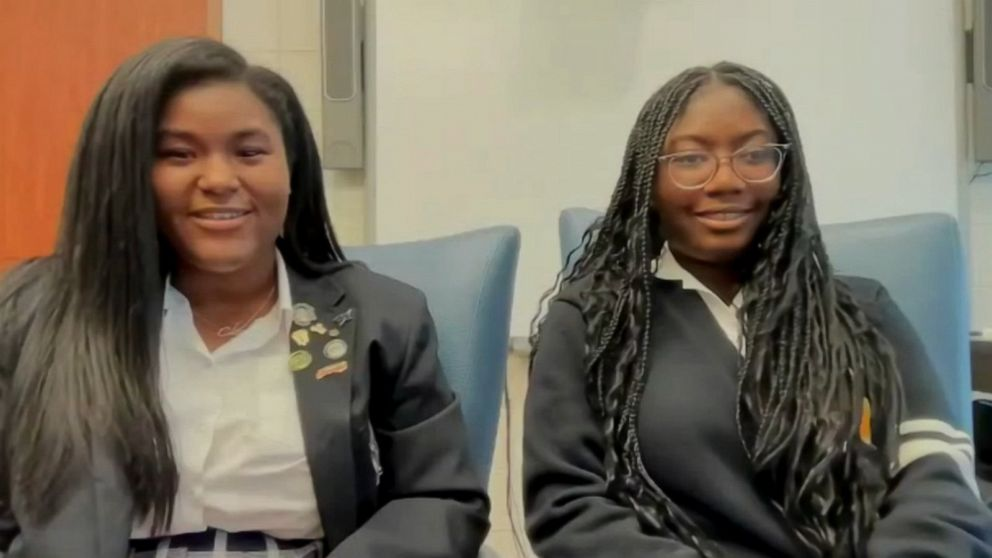# Two Black High School Students Might Be First To Find Trigonometric Proof

Two Black teenage students from New Orleans say they could be the first mathematicians in at least 2,000 years to find trigonometric proof for the Pythagorean TheoremTwo Black High School Students Might Be First To Find Trigonometric Proof

“After we get it peer reviewed and if it is approved, we would publish it in an undergraduate journal,” Calcea Johnson, one of the high school mathematicians, said. “And then it would be cemented in, pretty much, the math world.”

According to Johnson and her classmate, Ne’Kiya Jackson, the high school seniors of St. Mary’s Academy could be the first to use trigonometry to prove the Pythagorean Theorem.

The Pythagorean Theorem (a2 + b2 = c2) is usually taught in high school geometry and represents the theory that the two sides of a right triangle, when squared, equal the square of the hypotenuse, according to Johnson.

According to UCLA’s computer science department, scholars in ancient Babylon and Egypt knew of the theorem and it was displayed on a 4000-year-old Babylonian tablet. Pythagoras was an ancient Greek philosopher who revealed it to the western world nearly 2000 years later.